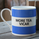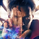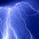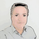‎54050‎ مشاهدة
54050
Revision: 1

Description:
• Strategy based around Open-Close Crossovers, which can be tuned for smooth trend trading, or with a little care even work for choppy waters .
Setup:
• I have generally found that setting the strategy resolution to 3-4x that of the chart you are viewing tends to yield the best results, regardless of which MA option you may choose (if any)
• Don't aim for perfection. Just aim to get a reasonably snug fit with the O-C band, with good runs of green and red.
• Option to either use basic open and close series data, or pick your poison with a wide array of MA types.
• Optional trailing stop for damage mitigation if desired (can be toggled on/off)
• Positions get taken directly following a crossover - which is why it's better to set the resolution of the script greater than that of your chart, so that the trades get taken sooner rather than later.
• If you make use of the trailing stops, be sure to take your time tweaking the values. Cutting it too fine may cost you profits but keep you safer, while letting them loose could lead to more drawdown than you can handle.
```//@version=2

strategy(title = "Open Close Cross Strategy", shorttitle = "OCC Strategy", overlay = true, pyramiding = 0, default_qty_type = strategy.percent_of_equity, default_qty_value = 10)

// Revision:        1
// Author:          @JayRogers
//
// Description:
//  - Strategy based around Open-Close Crossovers.
// Setup:
//  - I have generally found that setting the strategy resolution to 3-4x that of the chart you are viewing
//    tends to yield the best results, regardless of which MA option you may choose (if any)
//  - Don't aim for perfection. Just aim to get a reasonably snug fit with the O-C band, with good runs of
//    green and red.
//  - Option to either use basic open and close series data, or pick your poison with a wide array of MA types.
//  - Optional trailing stop for damage mitigation if desired (can be toggled on/off)
//  - Positions get taken automagically following a crossover - which is why it's better to set the resolution
//    of the script greater than that of your chart, so that the trades get taken sooner rather than later.
//  - If you make use of the trailing stops, be sure to take your time tweaking the values. Cutting it too fine
//    will cost you profits but keep you safer, while letting them loose could lead to more drawdown than you
//    can handle.

// === INPUTS ===
useRes      = input(defval = true, title = "Use Alternate Resolution? ( recommended )")
stratRes    = input(defval = "120", title = "Set Resolution ( should not be lower than chart )", type = resolution)
useMA       = input(defval = true, title = "Use MA? ( otherwise use simple Open/Close data )")
basisType   = input(defval = "DEMA", title = "MA Type: SMA, EMA, DEMA, TEMA, WMA, VWMA, SMMA, HullMA, LSMA, ALMA ( case sensitive )", type = string)
basisLen    = input(defval = 14, title = "MA Period", minval = 1)
offsetSigma = input(defval = 6, title = "Offset for LSMA / Sigma for ALMA", minval = 0)
offsetALMA  = input(defval = 0.85, title = "Offset for ALMA", minval = 0, step = 0.01)
useStop     = input(defval = true, title = "Use Trailing Stop?")
slPoints    = input(defval = 200, title = "Stop Loss Trail Points", minval = 1)
slOffset    = input(defval = 400, title = "Stop Loss Trail Offset", minval = 1)
// === /INPUTS ===

// === BASE FUNCTIONS ===
// Returns MA input selection variant, default to SMA if blank or typo.
variant(type, src, len, offSig, offALMA) =>
v1 = sma(src, len)                                                  // Simple
v2 = ema(src, len)                                                  // Exponential
v3 = 2 * v2 - ema(v2, len)                                          // Double Exponential
v4 = 3 * (v2 - ema(v2, len)) + ema(ema(v2, len), len)               // Triple Exponential
v5 = wma(src, len)                                                  // Weighted
v6 = vwma(src, len)                                                 // Volume Weighted
v7 = na(v5) ? sma(src, len) : (v5 * (len - 1) + src) / len    // Smoothed
v8 = wma(2 * wma(src, len / 2) - wma(src, len), round(sqrt(len)))   // Hull
v9 = linreg(src, len, offSig)                                       // Least Squares
v10 = alma(src, len, offALMA, offSig)                               // Arnaud Legoux
type=="EMA"?v2 : type=="DEMA"?v3 : type=="TEMA"?v4 : type=="WMA"?v5 : type=="VWMA"?v6 : type=="SMMA"?v7 : type=="HullMA"?v8 : type=="LSMA"?v9 : type=="ALMA"?v10 : v1
// security wrapper for repeat calls
reso(exp, use, res) => use ? security(tickerid, res, exp) : exp
// === /BASE FUNCTIONS ===

// === SERIES SETUP ===
// open/close
closeSeries = useMA ? reso(variant(basisType, close, basisLen, offsetSigma, offsetALMA), useRes, stratRes) : reso(close, useRes, stratRes)
openSeries  = useMA ? reso(variant(basisType, open, basisLen, offsetSigma, offsetALMA), useRes, stratRes) : reso(open, useRes, stratRes)
trendState  = closeSeries > openSeries ? true : closeSeries < openSeries ? false : trendState
// === /SERIES ===

// === PLOTTING ===
barcolor(color = closeSeries > openSeries ? #006600 : #990000, title = "Bar Colours")
// channel outline
closePlot   = plot(closeSeries, title = "Close Line", color = #009900, linewidth = 2, style = line, transp = 90)
openPlot    = plot(openSeries, title = "Open Line", color = #CC0000, linewidth = 2, style = line, transp = 90)
// channel fill
closePlotU  = plot(trendState ? closeSeries : na, transp = 100, editable = false)
openPlotU   = plot(trendState ? openSeries : na, transp = 100, editable = false)
closePlotD  = plot(trendState ? na : closeSeries, transp = 100, editable = false)
openPlotD   = plot(trendState ? na : openSeries, transp = 100, editable = false)
fill(openPlotU, closePlotU, title = "Up Trend Fill", color = #009900, transp = 40)
fill(openPlotD, closePlotD, title = "Down Trend Fill", color = #CC0000, transp = 40)
// === /PLOTTING ===

// === STRATEGY ===
// conditions
longCond    = crossover(closeSeries, openSeries)
shortCond   = crossunder(closeSeries, openSeries)
// entries and base exit
strategy.entry("long", strategy.long, when = longCond)
strategy.entry("short", strategy.short, when = shortCond)
// if we're using the trailing stop
if (useStop)
strategy.exit("XL", from_entry = "long", trail_points = slPoints, trail_offset = slOffset)
strategy.exit("XS", from_entry = "short", trail_points = slPoints, trail_offset = slOffset)
// not sure needed, but just incase..
strategy.exit("XL", from_entry = "long", when = shortCond)
strategy.exit("XS", from_entry = "short", when = longCond)
// === /STRATEGY ===```

## التعليقاتWARNING: For you newbies or those unfamiliar with Pine. This script does not work as well as you think. A quirk of Pine script means it will not perform like this real time.

JayRogers probably did not realise this when writing it.

EXPLANATION:

It gets data from a higher timeframe, i.e. your testing on a 1 minute chart pulling data from a 1 hour chart; "security(tickerid, res, exp) : exp"

A quirk of "//@version=2" of Pine in backtesting is when you pull data from a higher timeframe it looks to the close of that timeframe, i.e. on a 1 minute chart asking for a close price on the 1 hour chart, it will retrieve the close at the end of that hour. So in the 1st minute of the hour the script pulls the price at the end of the hour.

It effectively looks into the future, hence the excellent results.

TradingView have corrected this for "//@version=3". Change this to "//@version=3" and you get the true results.

People in here refer to this as "repaints".
ردrbarnesy
@rbarnesy, this is how to fix it.

trendState = false

changing this line to the below

trendState := closeSeries > openSeries ? true : closeSeries < openSeries ? false : trendState
ردyazeed
@yazeed, HI YAzeed, I have tried above yet not working, can you please may be paste the code here please.
ردJay nice work. Seems to yield great results right out of the box. A couple stupid questions for a new guy:
1. Stop Loss Trail Points: it defaults to 200 which I assume is 2% (yes/no)?
2. Stop Loss Tail Offset: defaults to 400... 400 what? Must be a dumb question I know...
Thanks!
PS. Can anyone chime in here? I would appreciate any clarity!!!
رد